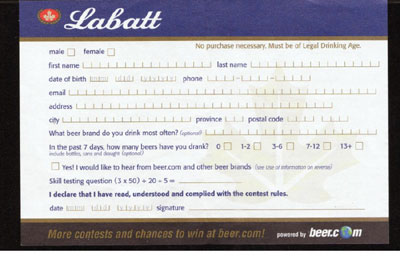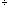Quandaries and Queries Allan you have to be 19 years old it is a contest Labatt Blue can you please give me the answer some people are saying 154 and some 34 Hi Allan,(350) + 205 The rules for the order in which we perform operations say we simplify within brackets first, then evaluate any exponents, then we perform multiplication and division (in the order in which they appear left to right) and then we perform addition and subtraction (in the order in which they apear left to right).  So in your question, only 20 is divided by 5, not the whole sum (350) + 20  and so the mathametically correct answer is 154. Leeanne These "skill testing" questions have always bothered me. I think that every one that I have seen is ambiguous. If they are going to use the rules of order that Leeanne quotes then why did they put parentheses around the "3 times 50"? The mathematically correct answer is what Leeanne says but I expect that the correct answer to the contest is whatever Labatt says it is. Harley Go to Math Central